# RS Aggarwal Class 9 Solutions Chapter 11 - Circle Ex 11C (11.3)

## RS Aggarwal Class 9 Chapter 11 – Circle Ex 11C (11.3) Solutions Free PDF

Students of Class 9 can clear their concepts by referring to the RS Aggarwal Class 9 solutions and practicing it on a regular basis will help them to prepare effectively for the exams. Scoring good marks in the exam is easy for the students with the help of reference RS Aggarwal Class solutions of Class 9 as it covers all the topics of the subject. Prepared by subject experts in accordance to the CBSE syllabus it also helps in improving the understanding of difficult concepts.

These solutions will make your foundation strong to tackle different variety of questions to practice in the most efficient manner. The RS Aggarwal Class 9 Solutions Chapter 11 – Circle Ex 11C (11.3) are easy to understand and crafted in such a way that all the steps of the solutions are described to match your understanding. Students are advised to solve RS Aggarwal maths solution which are highly useful for students.

## Download PDF of RS Aggarwal Class 9 Solutions Chapter 11 – Circle Ex 11C (11.3)

Question 1:In the given figure ,PQ is a diameter of a circle with centre O . If ∠ PRQ = 65°,∠ SPR = 40° and ∠ PQM = 50°    ,     find ∠QPR ,∠ QPM and ∠ PRS.

Sol.

Consider the triangle Δ PRQ.

PQ is the diameter.

The angle in a semicircle is a right angle.

⇒ ∠ PRQ = 90°

By the angle sum property in Δ PRQ, we have

∠QPR + ∠PRQ +∠PQR = 180°

⇒ ∠QPR + 90° + 65° = 180°

⇒ ∠QPR = 180° – 155° = 25° …….(1)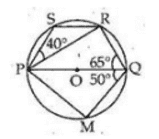Now Consider the triangle Δ PQM.

Since PQ is the diameter, ∠ PMQ = 90°’

Again by the angle sum property in Δ PRQ, we have

∠QPM+ ∠PMQ +∠PQM = 180°

⇒ ∠QPM + 90° + 50° = 180°

⇒ ∠QPR = 180° – 140° = 40°

Now in quadrilateral PQRS

∠QPS + ∠ SRQ = 180°

⇒ ∠QPR+ ∠RPS +∠PRQ + ∠ PRS = 180° [from (1)]

⇒ 25° + 40° + 90° + ∠ PRS = 180°

⇒       ∠ PRS = 180° – 155° = 25°

∴      ∠ PRS = 25°

Question 2: In the given figure ABCD is a cyclic quadrilateral whose diagonals intersect at P such that ∠ DBC = 60° and ∠ BAC = 40° , find

1. ∠ BCD

Sol.

(i) ∠ BDC = ∠ BAC = 40° [angles in the same segment]

In Δ BCD, we have

∠ BCD +∠ BDC + ∠ DBC =180°

∴ ∠ BCD +40° + 60° =180°

⇒ ∠ BCD = 180° -100° = 80°

∴ ∠ BCD = 80°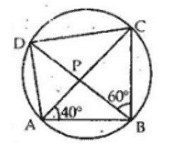(ii) Also ∠ CAD = ∠ CBD [angles in the same segment]

∠ CAD = 60° [∵ ∠ CBD = 60°]

Question 3: In the given figure, PQR is a diameter and PQRS is a cyclic quadrilateral. If ∠ PSR = 150°, find∠ RPQ

Sol.

In cyclic quadrilateral PQRS

∠ PSR + ∠ PQR = 180°

⇒ 150° + ∠ PQR = 180°

⇒ ∠ PQR = 180° – 150° = 30° …….(1)

Also, ∠ PQR = 90° …….(2) [angles in a semicircle]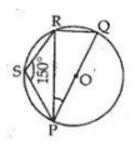Now in Δ PRQ, we have

∠ PQR + ∠ PRQ + ∠ RPQ = 180°

⇒ 30° + 90°+ ∠ RPQ = 180° [from (i) and (ii)]

⇒ ∠ RPQ = 180° – 120° = 60°

∴ ∠ RPQ = 60°

Question 4: In the given figure ABCD is a cyclic quadrilateral in which AB || DC . If       ∠ BAD = 100°  , find

1. ∠ BCD
3. ∠ ABC

Sol.

In cyclic quadrilateral ABCD, AB || DC and ∠ BAD = 100°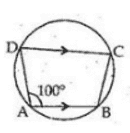(i) ∠ BCD + ∠ BAD = 180°

⇒ ∠ BCD + 100° = 180°

⇒ ∠ BCD = 180° – 100° = 80°

(ii) Also, ∠ ADC = ∠ BCD = 80°

∴ ∠ ACD = 80°

(iii) ∠ ABC = ∠ BAD = 100°

∠ ABC = 100°

Question 5: In the given figure O is the centre of the circle and arc ABC subtends an angle of 1300 at the centre . If  AB is extended to P , find ∠ PBC

Sol.

Take a point D on the major arc CA and join AD and DC

∴ ∠ 2 = 2∠ 1 [Angle subtended by an arc is twice the angle subtended by it on the circumference in the alternate segment.]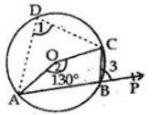∴ 130° = 2∠ 1

⇒ ∠ 1 = 65° …….(1)

∠ PBC = ∠1 [∵ Exterior angle of a cyclic quadrilateral interior opposite angle]

∴ ∠ PBC = 65°

Question 6: In the given figure ABCD is a cyclic quadrilateral in which AE is drawn parallel to CD and BA is produced . If∠ABC=92°  and ∠ EAF = 20°, find∠ BCD.

Sol.

ABCD is acyclic quadrilateral

∴ ∠ABC + ∠ ADC = 180°

⇒ 92° + ∠ ADC = 180°

⇒ ∠ ADC = 180° -92° = 88°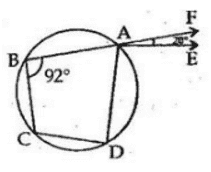Also, AE || CD

∴ ∠EAD = ∠ ADC = 88°

∴ ∠ BCD = ∠ DAF [∵ Exterior angle of a cyclic quadrilateral = interior opposite angle]

∴ ∠ BCD = ∠ EAD + ∠ EAF

= 88° + 20° [∵∠ FAE = 20° (given)]

= 108°

∴ ∠ BCD = 108°

Question 7: In the given figure , BD=DC and ∠ CBD = 30°, find m(∠ BAC)

Sol.

BD = DC

∴ ∠ BCD = ∠ CBD = 30°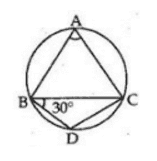In Δ BCD, we have

∠ BCD + ∠ CBD + ∠ CDB = 180°

⇒ 30° + 30° + ∠ CDB = 180°

⇒ ∠ CDB = 180° – 60°

= 120°

The opposite angles of a cyclic quadrilateral are supplementary.

ABCD is a cyclic quadrilateral and thus,

∠ CDB + ∠ BAC = 180°

= 180° -120° [∵∠ CDB = 120°]

= 60°

∴ ∠ BAC = 60°

Question 8: In the given figure , O is the centre of the given circle and measure of arc ABC is 1000. Determine ∠ ADC  and ∠ ABC .

Sol.

Angle subtended by an arc is twice the angle subtended by it on the circumference in the alternate segment.

Here arc ABC makes ∠ AOC = 100° at the center of the circle and ∠ ADC on the circumference of the circle.

∴ ∠ AOC = 2∠ ACD

⇒ ∠ ACD = ½ (∠AOC)

⇒ = ½ × 100° [∠ AOC = 100°]

⇒ ∠ ACD = 50°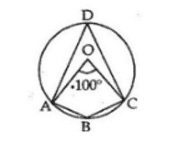The opposite angles of a cyclic quadrilateral are supplementary.

ABCD is a cyclic quadrilateral and thus,

∠ ACD + ∠ ABC = 180°

= 180° – 50° [∵ ∠ ADC = 50°]

= 130°

∴ ∠ ABC = 130°

∴ ∠ ACD = 50° and ∠ ABC = 130°

Question 9: In the given figure , Δ ABC is equilateral . Find

1. ∠ BDC
2. ∠ BEC

Sol.

Δ ABC is an equilateral triangle.

∴   Each of its angle is equal to 60°

⇒ ∠ BAC = ∠ ABC = ∠  ∠ ACB = 60°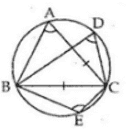(i) Angles in the same segment of a circle are equal.

∴ ∠ BDC = ∠ BAC

= 60° [∵ ∠ BAC = 60°]

(ii) The opposite angles of a cyclic quadrilateral are supplementary.

ABCE is a cyclic quadrilateral and thus,

∠ BAC + ∠ BEC = 180°

∠ BEC = 180° – 60° [∵ ∠ BAC = 60°]

= 120°

⇒ ∠ BEC = 120°

Question 10: In the adjoining figure, ABCD is a cyclic quadrilateral in which ∠ BCD = 100° and ∠ ABD = 50° , find ∠ ADB

Sol.

ABCD is a cyclic quadrilateral,

∠ A + ∠ C = 180° [The opposite angles of a cyclic quadrilateral are supplementary]

⇒ ∠ A + 100°= 180°

⇒ ∠ A = 180° – 100° = 80°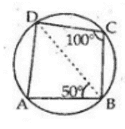Now in Δ ABD, we have

∠ A + ∠ ABD + ∠ ADB = 180°

⇒ 80° + 50° + ∠ ADB = 180°

⇒ ∠ ADB = 180° – 130° = 50°

∴ ∠ ADB = 50°

Question 11: In the given figure , O is the centre of the circle and ∠ DAB = 150° find value of x and y

Sol.

O is the center of the circle and ∠ BOD = 150°

∴ Reflex   ∠ BOD = (360° – ∠ BOD)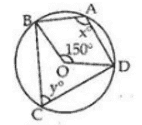= (360° – 150°) = 210°

Now, X = ½(reflex ∠ BOD)

= ½ × 210° = 105°

X = 105°

Again, X + Y = 180°

⇒ 105° + Y = 180°

⇒           Y = 180° – 105° = 75°

∴            Y = 75°

Question 12: In the given figure , O is the centre of the circle and ∠ DAB = 50° . Find value of x and y.

Sol.

O is the center of the circle and ∠ DAB= 50°

OA = OB [Radii]

⇒ ∠ OBA = ∠ OAB = 50°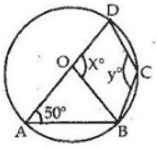In Δ OAB, we have

∠ OAB + ∠ OBA + ∠ AOB =180°

⇒   50° + 50° + ∠ AOB =180°

⇒    ∠ AOB =180° – 100° = 80°

Since, AOD is a straight line,

X = 180° – ∠ AOB

= 180° – 80° = 100°

∴ X = 100°

The opposite angles of a cyclic quadrilateral are supplementary.

ABCD is a cyclic quadrilateral and thus,

∠ DAB + ∠ BCD = 180°

∠ BCD = 180° – 50° [∵ ∠ DAB = 50°, given]

⇒ Y = 130°

Thus, X = 100° and Y = 130°.

Question 13: In the given figure sides AD and AB of cyclic quadrilateral ABCD are produced to E and F respectively. If ∠ CBF=130°  and ∠ CDE= X0 , find the value of x

Sol.

ABCD is a cyclic quadrilateral

We know that in a cyclic quadrilateral exterior angle = interior angle.

∴ ∠ CBF = ∠ CDA = (180° – X)

⇒ 130° = 180° – X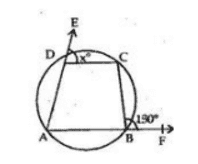⇒ X = 180° – 130° = 50°

X = 50°

### Key Features of RS Aggarwal Class 9 Solutions Chapter 11 – Circle Ex 11C (11.3)

• It will improve your mathematical problem-solving skills.
• It gives ample techniques to solve difficult and tough questions.
• It is the best reference study material.
• It helps in boosting up your confidence.